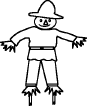EnchantedLearning.com is a user-supported site.
As a bonus, site members have access to a banner-ad-free version of the site, with print-friendly pages.

 Go to the AnswersMath Pages EnchantedLearning.com Divide Twelve Fall Items Divide the fall items into groups to do division. Visual Division PagesDivision Pages

 Divide the scarecrows into two equal groups.How many scarecrows are there? How many groups of scarecrows are there? How many scarecrows are in each group?
12 ÷ 2 =

 Divide the leaves into three equal groups.How many leaves are there? How many groups of leaves are there? How many leaves are in each group?
12 ÷ 3 =

 Divide the rakes into six equal groups.How many rakes are there? How many groups of rakes are there? How many rakes are in each group?
12 ÷ 6 =

## Enchanted Learning Search

 Search the Enchanted Learning website for: